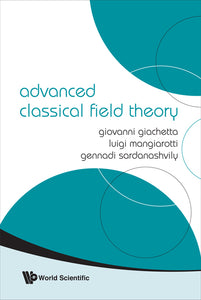Giovanni Giachetta

Format: Print Book

ISBN: 9789812838957

• \$149.85
Unit price per
Tax included.

Contemporary quantum field theory is mainly developed as quantization of classical fields. Therefore, classical field theory and its BRST extension is the necessary step towards quantum field theory. This book aims to provide a complete mathematical foundation of Lagrangian classical field theory and its BRST extension for the purpose of quantization. Based on the standard geometric formulation of theory of nonlinear differential operators, Lagrangian field theory is treated in a very general setting. Reducible degenerate Lagrangian theories of even and odd fields on an arbitrary smooth manifold are considered. The second Noether theorems generalized to these theories and formulated in the homology terms provide the strict mathematical formulation of BRST extended classical field theory. The most physically relevant field theories — gauge theory on principal bundles, gravitation theory on natural bundles, theory of spinor fields and topological field theory — are presented in a complete way.This book is designed for theoreticians and mathematical physicists specializing in field theory. The authors have tried throughout to provide the necessary mathematical background, thus making the exposition self-contained.

Format: Hardcover
No of Pages: 392
Imprint: World Scientific
Publication date: 20090511